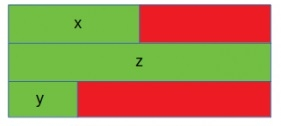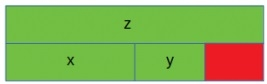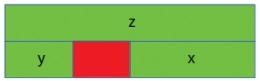# Why isn't sizeof for a struct equal to the sum of sizeof of each member in C/C++?

The size of a struct type element taken by sizeof() is not always equal to the size of each individual member. Sometimes the compilers add some padding to avoid alignment issues. So the size may change. The padding is added when a structure member is followed by a member with a larger size or at the end of the structure. Different compiler has different types of alignment constraints. In C standard, the total alignment structure depends on the implementation.

## Case 1

In this case the double z is 8-byte long, which is larger than x (4-byte). So another 4-byte padding is added. Also short type data y has 2-byte space in memory so extra 6-bytes are added as padding.## Example Code

#include <stdio.h>
struct myStruct {
int x; //Integer takes 4 bytes, and padding 4 bytes
double z; //Size of double is 8-byte, no padding
short int y; //Size of short is 2-byte, padding 6-bytes
};
main() {
printf("Size of struct: %d", sizeof(struct myStruct));
}

## Output 2

Size of struct: 24

## Case 2

In this case the double is inserted at first, and it takes 8-bytes of space. Now the integer x (4-byte) is added. So there is another 4-byte space. When the short y is added, it can be placed into that extra 4-byte space and occupies total 16-bytes of space.## Example Code

#include <stdio.h>
struct myStruct {
double z; //Size of double is 8-byte, no padding
int x; //Integer takes 4 bytes, and padding 4 bytes
short int y; //Size of short is 2-byte, padding 6-bytes
};
main() {
printf("Size of struct: %d", sizeof(struct myStruct));
}

## Output 2

Size of struct: 16

## Case 3

In the third case it also takes 16-byte of memory space, but the arrangements are different. As the first member is double then it is placed at first, then the short type data is added. Now when the integer is trying to insert, it can be placed into the remaining 6-byte area. So one padding is present after short but no padding is required after the integer data.## Example Code

#include <stdio.h>
struct myStruct {
double z; //Size of double is 8-byte, no padding
short int y; //Size of short is 2-byte, padding 6-bytes
int x; //Integer takes 4 bytes, and padding 4 bytes
};
main() {
printf("Size of struct: %d", sizeof(struct myStruct));
}

## Output 2

Size of struct: 16